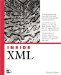# Recipe 8.10 Creating Nonrectangular Windows

### Recipe 8.10 Creating Nonrectangular Windows

#### 8.10.1 Problem

You want to wow users with windows of arbitrary shapes , using them to display company logos, game pieces, advertisements, and so on.

#### 8.10.2 Solution

Create a nonrectangular shell using shell regions in Eclipse 3.0. In Eclipse 3.0, SWT shells can now have an irregular shape defined by regions . As an example ( NonRectangularApp at this book's site), we'll create a blue doughnut-shaped window with an Exit button in it. All you have to do is to create a shell, create a Region object that defines the shape you want to use, and use the shell's setRegion method to configure the shell.

#### 8.10.3 Discussion

In this example, we'll use a method named createCircle to create circular regions. The following example shows how to use that method and those regions to create a doughnut-shaped shell that we'll color blue using the shell's setBackground method. Note that you can add and cut regions from the final shell using the Region class's add and subtract methods :

` final Display display = new Display( ); final Shell shell = new Shell(display, SWT.NO_TRIM);  Region region = new Region( );   region.add(createCircle(50, 50, 50));   region.subtract(createCircle(50, 50, 20));   shell.setRegion(region);   shell.setSize(region.getBounds( ).width, region.getBounds( ).height);   shell.setBackground(display.getSystemColor(SWT.COLOR_BLUE));  . . . `

To create circles, the createCircle method returns an array of points outlining the circle. Here's how this method does that:

` static int[] createCircle(int xOffset, int yOffset, int radius) {     int[] circlePoints = new int[10 * radius];     for (int loopIndex = 0; loopIndex < 2 * radius + 1; loopIndex++) {         int xCurrent = loopIndex - radius;         int yCurrent = (int) Math.sqrt(radius * radius                - xCurrent * xCurrent);         int doubleLoopIndex = 2 * loopIndex;                  circlePoints[doubleLoopIndex] = xCurrent + xOffset;         circlePoints[doubleLoopIndex + 1] = yCurrent + yOffset;         circlePoints[10 * radius - doubleLoopIndex - 2] = xCurrent + xOffset;         circlePoints[10 * radius - doubleLoopIndex - 1] = -yCurrent                + yOffset;     }          return circlePoints; } `

All that's left is to create an Exit button and add it to the code, which you can see in Example 8-8.

##### Example 8-8. Creating nonrectangular windows
` package org.cookbook.ch08; import org.eclipse.swt.*; import org.eclipse.swt.graphics.*; import org.eclipse.swt.widgets.*; public class NonRectangularClass {          static int[] createCircle(int xOffset, int yOffset, int radius) {         int[] circlePoints = new int[10 * radius];         for (int loopIndex = 0; loopIndex < 2 * radius + 1; loopIndex++) {             int xCurrent = loopIndex - radius;             int yCurrent = (int) Math.sqrt(radius * radius - xCurrent                    * xCurrent);             int doubleLoopIndex = 2 * loopIndex;                          circlePoints[doubleLoopIndex] = xCurrent + xOffset;             circlePoints[doubleLoopIndex + 1] = yCurrent + yOffset;             circlePoints[10 * radius - doubleLoopIndex - 2] = xCurrent + xOffset;             circlePoints[10 * radius - doubleLoopIndex - 1] = -yCurrent                    + yOffset;         }                  return circlePoints;     }          public static void main(String[] args) {         final Display display = new Display( );         final Shell shell = new Shell(display, SWT.NO_TRIM);                  Region region = new Region( );         region.add(createCircle(50, 50, 50));         region.subtract(createCircle(50, 50, 20));         shell.setRegion(region);         shell.setSize(region.getBounds( ).width, region.getBounds( ).height);         shell.setBackground(display.getSystemColor(SWT.COLOR_BLUE));  sButton button = new Button(shell, SWT.PUSH);         button.setText("Exit");         button.setBounds(35, 6, 35, 20);         button.addListener(SWT.Selection, new Listener( ) {             public void handleEvent(Event event) {                 shell.close( );             }         });  shell.open( );         while (!shell.isDisposed( )) {             if (!display.readAndDispatch( ))                 display.sleep( );         }         region.dispose( );         display.dispose( );     } } `

That's all you need; you can see the results in Figure 8-9. Very cool.

##### Figure 8-9. A doughnut-shaped windowInside XML (Inside (New Riders))
ISBN: 596007108
EAN: 2147483647
Year: 2006
Pages: 232
Authors: Steve Holzner